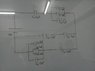# Individual Charges and voltage

Shiba Tatsuya

## Homework Statement

the capacitance of each capacitors and the cell voltage

## The Attempt at a Solution

I got the total capacitance = 8028/5333 uF
total voltage = 36v
total charge=289008/5333 uC

#### Attachments

•25130120_1726398060724048_1055864124_o.jpg
28.2 KB · Views: 375

Gold Member
You should systematically split the total capacitance and the total charge. Each time there should be a simultaneous equation (one equation of capacitance and the other of charge).

I have a feeling I am suggesting the unnecessarily long way, there might be a shorter method.

Shiba Tatsuya
You should systematically split the total capacitance and the total charge. Each time there should be a simultaneous equation (one equation of capacitance and the other of charge).

I have a feeling I am suggesting the unnecessarily long way, there might be a shorter method.

but how? the at the top wiring it is a series connection but at the middle and the bottom, it is parallel :/

Gold Member
but how? the at the top wiring it is a series connection but at the middle and the bottom, it is parallel :/
But you still know the equations for both. For know, combine anything parallel and make it series. Later, you can split them into parallel again by using the same backwards technique.

Let me get you started. Solve for the top part of the circuit and the two bottom parts combined. Then split the two bottom parts into separate parts. You will have three capacitances of three parts. You can continue.

Shiba Tatsuya
But you still know the equations for both. For know, combine anything parallel and make it series. Later, you can split them into parallel again by using the same backwards technique.

Let me get you started. Solve for the top part of the circuit and the two bottom parts combined. Then split the two bottom parts into separate parts. You will have three capacitances of three parts. You can continue.

by splitting, you mean dividing the result into two?

Gold Member
by splitting, you mean dividing the result into two?
No, I mean going backward. Think about how the charge changes when you add parallely and how the capacitance changes when you add parallely. Then work backwards with the two equations.

For example, say I have the total capacitance and charge of a parallel circuit with two sections, C and Q. The upper section has capacitance and charge, C1 and Q1. The lower section has capacitance and charge, C2 and Q2. I know that Q1 + Q2 = Q and the same can be said for capacitance, because capacitances and charges add together when in parallel. Knowing that Q = CV, you can solve for the necessary variables.

Shiba Tatsuya
No, I mean going backward. Think about how the charge changes when you add parallely and how the capacitance changes when you add parallely. Then work backwards with the two equations.

For example, say I have the total capacitance and charge of a parallel circuit with two sections, C and Q. The upper section has capacitance and charge, C1 and Q1. The lower section has capacitance and charge, C2 and Q2. I know that Q1 + Q2 = Q and the same can be said for capacitance, because capacitances and charges add together when in parallel. Knowing that Q = CV, you can solve for the necessary variables.

thank you :D I also noticed this relationship :D I'm done now :D

Gold Member
thank you :D I also noticed this relationship :D I'm done now :D